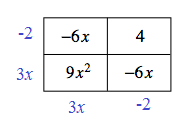### Home > CCA > Chapter 7 > Lesson 7.2.3 > Problem7-109

7-109.

Find the dimensions of the generic rectangle shown at right and write its area as a sum and a product. Homework Help ✎

 $-6x$ $4$ $9x^2$ $-6x$

To express the area as a product, find the dimensions of the large rectangle and multiply them together. To express the area as a sum, add the tile pieces together.